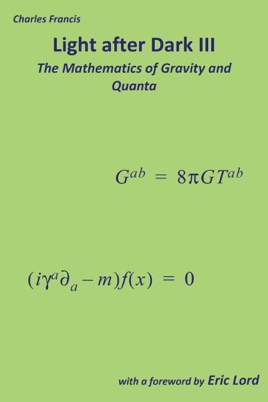• \$9.99

Publisher Description

Light after Dark III: The Mathematics of Gravity and Quanta proves the most important results described in Light after Dark II, from fundamental principles, as elegantly as possible and with the minimum of verbiage. This is a first principles account of the mathematical structure of modern theoretical physics, showing that it is not just a disparate bunch of algorithms and procedures, but is a unified structure based on observation, sound principles and solid logic, and allowing a unique physical interpretation in terms of fundamental constituents, or particles.

Light after Dark III: The Mathematics of Gravity and Quanta explains essential mathematics at undergraduate level. Part I covers many core topics in a mathematics degree. Part II is a concise account of results in relativity, including the Lorentz transform from first principles, Riemann curvature, Einstein’s equation, derivations of the Schwarzschild and Friedmann solutions, and proofs of key results. Part III contains the mathematical foundations of relativistic quantum mechanics and constructs quantum electrodynamics. Divergence problems are treated with greater rigour than usual in theoretical physics, but without excessive formality. Classical electromagnetism is derived from fundamental principles. Part IV shows how quantum electrodynamics and general relativity can be described in a single structure.

Light after Dark III: The Mathematics of Gravity and Quanta will interest students of mathematics and students of physics and philosophy with a mathematical leaning who are seeking a rigorous treatment and deeper mathematical insight into physical theory.